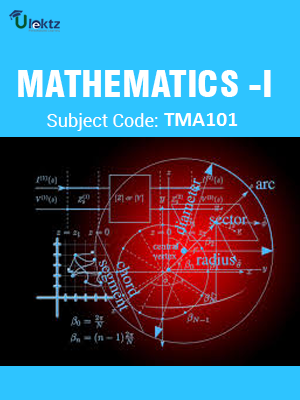•My WalletMy Order
•My Profile
•My Connections
•My Books
•My Videos
•My Tests
•My Calender
•My Messages
•My Shopping Cart
•My Orders
•Account Settings
•Help

# Book Details# MATHEMATICS-I

 Course Code : TMA101 Author : uLektz University : Uttarakhand Technical University Regulation : 2010 Categories : Engineering Mathematics Format :ePUB3 (DRM Protected) Type : eBook

FREE

Description :MATHEMATICS-I of TMA101 covers the latest syllabus prescribed by Uttarakhand Technical University for regulation 2010. Author: uLektz, Published by uLektz Learning Solutions Private Limited.

Note : No printed book. Only ebook. Access eBook using uLektz apps for Android, iOS and Windows Desktop PC.

##### Topics
###### UNIT - I MATRICES

1.1 Elementary row and column transformations, Rank of matrix

1.2 Linear dependence, Consistency of linear system of equations, Characteristic equation

1.3 Cayley-Hamilton theorem

1.4 Eigen values and Eigen vectors, Diagonalization

1.5 Complex and unitary matrices

###### UNIT - II DIFFERENTIAL CALCULUS - I

2.1 Leibnitz theorem

2.2 Partial Differentiation

2.3 Euler’s theorem, Change of variables, Expansion of functions of several variables

###### UNIT - III DIFFERENTIAL CALCULUS - II

3.1 Jacobian

3.2 Approximations and errors, Extrema of functions of several variables

3.3 Lagrange method of multipliers

###### UNIT - IV MULTIPLE INTEGRALS

4.1 Double and triple integrals, Change of order, Change of variables

4.2 Beta and gamma functions, Application to area, volume

4.3 Dirichlet integral and applications

###### UNIT - V VECTOR CALCULUS

5.1 Point functions, Gradient, divergence and curl of a vector and their physical interpretation

5.2 Line, surface and volume integrals

5.3 Green’s theorem

5.4 Stokes theorem

5.5 Gauss divergence theorem